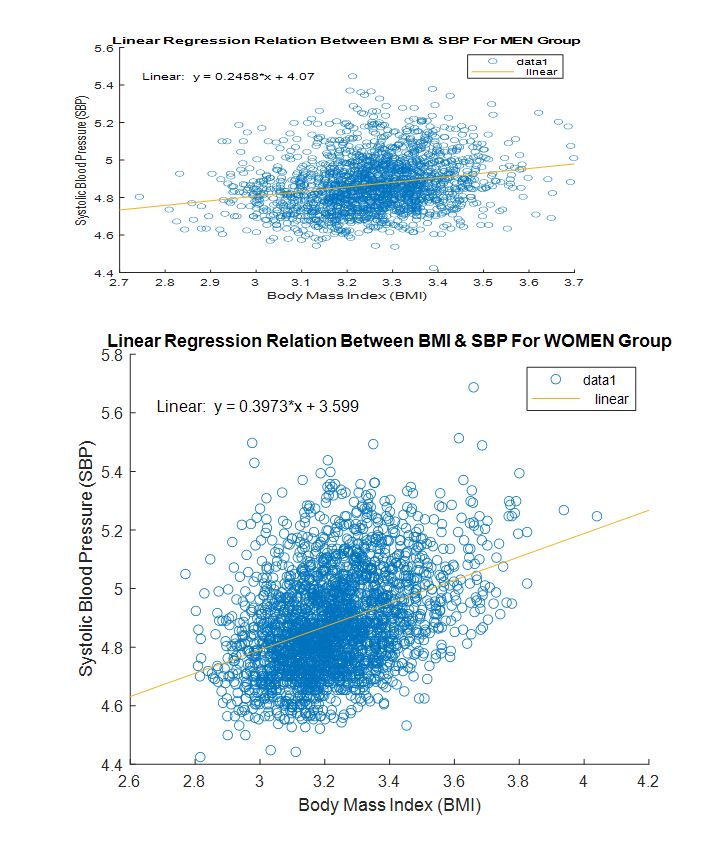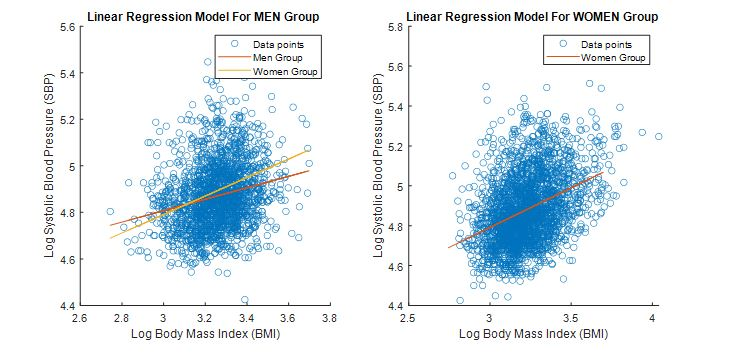# Professional Data Analysis Assignment Help Using MATLAB

Impress your professor with well-written and correct solutions by taking our data analysis assignment help using MATLAB. Our service is all about excellent solutions at an affordable rate. The only thing you will regret when you avail of our data analysis homework help using MATLAB is not coming to us sooner. Our competent and trustworthy MATLAB assignment experts have helped several students to secure academic excellence. Your case will not be any different. Place your order now.

## Data Analysis Using MATLAB

Write a MATLAB script to solve the following questions. Insert figures, tables, and answers directly into this document.
Background
The Framingham Heart Study (Levy, 1999) has collected cardiovascular risk factor data and long-term follow-up on almost 5000 residents of the town of Framingham, Massachusetts. Refer to the “Framingham Documentation” pdf file for detailed information on the data set. These questions are modeled after analyses in DOI:10.1017/CBO9780511575884.

## Linear Regression

Perform linear regression to show the relationship between systolic blood pressure (SBP) and body mass index (BMI).
An exploratory analysis and assumption-checking indicated that the relationship between log(SBP) and log(BMI) comes closer to meeting the assumptions of a linear model than does the relationship between SBP and BMI .
1. Create two regression models. Regress log(SBP)against log(BMI) for men and women. Note that SBP is the variable plotted on the y-axis and BMI is on the x-axis.
Fill in the following table with your results:
Table 1. Regression Coefficients for linear models.

 Sex Number of subjects ni β0 β 1 Men 1194 4.07 0.2458 Women 2490 3.599 0.3973## Using the Function ‘coefCI’to Find a 95% CI on β 1 Values

Table 2. Confidence intervals on β 1 values

 Sex Lower Bound Upper Bound Men 0.1993 0.2923 Women 0.3599 0.4347
3. Use these CIs to determine if the slopes of the two best-fit lines are equal.

Answer: The slopes of the two best-fit are not equal.
4. Use your models to predict the following:
a. SBP of a man with BMI=33.
Answer: The linear model equation for men is calculated as: y=0.2458x+4.07. For BMI=33, the SBP=12.1814
b. SBP of a woman with BMI=33.
Answer: The linear model equation for women is calculated as: y=0.3973x+3.599. For BMI=33, the SBP=16.71
5. Plot both sets of data. Use the subplot command to include them both in a single figure. The left-hand subplot should contain a log-log scale plot of the data for men with two regression lines, one for men and one for women(to highlight any differences in slope). The right-hand subplot should contain a log-log scale plot of the data for women with the regression line for women. Make sure that your regression line is linear.## Multiple Regression Model

We will now create a multiple regression model that seeks to predict SBP based on additional variables included in the Framingham heart study.
A first step is to create several linear models of various variables that are possibly related to SBP. We will not be using the log transform for these models. Make three separate simple regression models:
a. SBP against BMI– do NOT separate the data by sex.
b. SBP against age
c. SBP against serum cholesterol
Fill in the following table:
Table 3. Results of three separate linear regression models.
 Model β 1 P-value for β 1 R2 a 1.7885 0.0000 0.1076 b 1.0297 0.0000 0.1588 c 0.1004 0.0000 0.0400
d. Comment on the significance of regression of these models.
Answer: For all three models, the p-value of 0.0000 is less than the default significance level of 0.05, however the R2 values is far less to 1, a significant linear regression relationship does not exist between the response Y and the predictor variables in X.
6. Note that each individual model’s R2 value is low, indicating that no single variable is doing a good job of explaining the total variability in the data. A multivariate model may address this issue and provide better predictions. Create a multiple regression model of SBP against BMI, age, and serum cholesterol.
Fill in the following table:
Table 4. Estimated coefficients for multiple regression model.
 Estimate SE tStat pValue Intercept 40.8364 0.2407 460.6981 0.0000 BMI 1.4945 Age 0.8749 SCL 0.0412

7. Comment on the significance of regression for each of the three regressors.

Answer: After using multiple regression model, BMI has the more effect on the SBP compared to Age and SCL.

8. Predict the value of SBP for an individual with a BMI of 33, an age of 55 years, and a cholesterol level of 288 mg/dL. Include a 95% prediction interval.

Answer: The equation after multiple linear regression is came out to be: SBP=40.8364+1.4945xBMI+0.8749xAge+0.0412xSCL. After putting the values, we get SBP=150.14.

Extra Credit: Create a new multiple regression model to predict SBP using the Framingham data set. All regressors must be significant and your adjusted R2must be above 0.25 to get credit. Show the results of your model in a table similar to table 4.

Analysis 1 Solution

clc

clear all

close all

%%%%%%%%% Import Framingham data

data=data{:,:}; %%%% converting table into matrix form

data2=data(1:4434,:); %%%% selecting data for Period 1 only

data3=sortrows(data2,2); %%%% sorting data based on gender

%%

%%%%%%% For men

X=[ones(size(BMI_men)) BMI_men]; %%%% making X matrix for the linear regression model

Y=SBP_men;

[b_men,bint_men]=regress(Y,X) %%%% applying linear regression model to find coefficients and CIs

%%%%% using model to predict SBP values for men

x_men=33;

y_men=0.2458*x_men+4.07

%%

%%%%%%% For WOMEN

X=[ones(size(BMI_women)) BMI_women]; %%%% making X matrix for the linear regression model

Y=SBP_women;

[b_women,bint_women]=regress(Y,X) %%%% applying linear regression model to find coefficients and CIs

%%%%% using model to predict SBP values for women

x_women=33;

y_women=0.3973*x_women+3.599

%%

%%%%%% ploting

subplot(1,2,1)

yCalc1_men1=0.2458.*BMI_men+4.07;

yCalc1_women1=0.3973.*BMI_men+3.599;

scatter(BMI_men,SBP_men)

hold on

plot(BMI_men,yCalc1_men1)

hold on

plot(BMI_men,yCalc1_women1)

xlabel('Log Body Mass Index (BMI)')

ylabel('Log Systolic Blood Pressure (SBP)')

title('Linear Regression Model For MEN Group')

legend('Data points','MenGroup','Women Group')

subplot(1,2,2)

scatter(BMI_women,SBP_women)

hold on

hold on

plot(BMI_men,yCalc1_women1)

xlabel('Log Body Mass Index (BMI)')

ylabel('Log Systolic Blood Pressure (SBP)')

title('Linear Regression Model For WOMEN Group')

legend('Data points','Women Group')

Analysis 2 Solution

clc

clear all

close all

%%%%%%%%% Import Framingham data

data=data{:,:}; %%%% converting table into matrix form

data2=data(1:4434,:); %%%% selecting data for Period 1 only

%%

%%%%%% Model 1: SBP against BMI without separating the data by sex

X1=[ones(size(BMI_1)) BMI_1]; %%%% making X matrix for the linear regression model

Y1=SBP_1;

[b1,~,~,~,stats_1]=regress(Y1,X1) %%%% applying linear regression model to find coefficients

%%

%%%%%% Model 2: SBP against age

X2=[ones(size(AGE_2)) AGE_2]; %%%% making X matrix for the linear regression model

Y2=SBP_2;

[b2,~,~,~,stats_2]=regress(Y2,X2) %%%% applying linear regression model to find coefficients

%%

%%%%%% Model 3: SBP against serum cholesterol

X3=[ones(size(TOTCHOL_3)) TOTCHOL_3]; %%%% making X matrix for the linear regression model

Y3=SBP_3;

[b3,~,~,~,stats_3]=regress(Y3,X3) %%%% applying linear regression model to find coefficients

%%

%%%%%% Multivariate Linear Regression Model

%%%%%% Multivariate Model: SBP against serum cholesterol